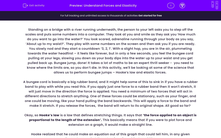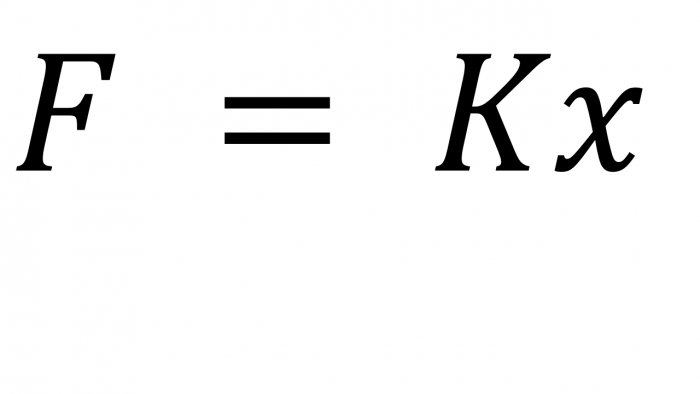# Understand Forces and Elasticity

In this worksheet, students will learn Hooke's law, applying the equation and graph.Key stage:  KS 4

Year:  GCSE

GCSE Boards:   OCR 21st Century, Pearson Edexcel, AQA, AQA Trilogy, AQA Synergy, OCR Gateway, Eduqas,

Popular topics:   Physics worksheets

Difficulty level:#### Worksheet Overview

A bungee cord is basically a big rubber band, and it might help some of this to sink in if you have a rubber band to play with while you read this. If you apply just one force to a rubber band then it won't stretch, it will just move in the direction the force is applied. You need a minimum of two forces that will act in different directions to stretch anything. One of these forces could be stationary, such as your finger, and one could be moving, like your hand pulling the band backwards. This will apply a force to the band and make it stretch. If you release the forces,  the band will return to its original shape. All good so far?

Okay, so Hooke’s law is a law that defines stretching things. It says that ‘the force applied to an object is proportional to the length of the extension’. This basically means that if you were to plot force and extension on a graph, it would make a straight line.

Hooke realized that he could make an equation out of this graph that could tell him, in any given circumstance, what the extension of an object would be.F = force (newtons (N))
k = spring constant – a number that tells you how hard it is to stretch the object (newtons per metre (N/m))
x = extension – how much the spring has stretched (metres (m)) This is often also written as e for extension and this is what we will be using in this activity.

So, the person putting the numbers into the computer at the start of the activity was just working out how much you would stretch the bungee, using this equation.

Let’s have a go at this ourselves.

Question:  A person stretches the bungee 15 m when the bungee cord has a spring constant of 40 N/m. Calculate the force applied by that person.

Step 1   Highlight the numbers in the question:

A person stretches the bungee 15 m when the bungee cord has a spring constant of 40 N/m. Calculate the force applied by that person.

Step 2   Write out the numbers:
F = ?
k = 40 N/m
e = 15 m

Step 3 Put the numbers into the equation:

F = 40 x 15

F = 600 N

Let's have a go at some questions now.

### What is EdPlace?

We're your National Curriculum aligned online education content provider helping each child succeed in English, maths and science from year 1 to GCSE. With an EdPlace account you’ll be able to track and measure progress, helping each child achieve their best. We build confidence and attainment by personalising each child’s learning at a level that suits them.

Get started#### Popular Science topics

••••